# High School Math : Law of Cosines

## Example Questions

### Example Question #1 : Applying The Law Of Cosines

In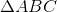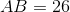,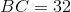, and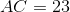. To the nearest tenth, what is?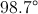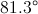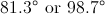A triangle with these sidelengths cannot exist.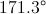Explanation:

By the Triangle Inequality, this triangle can exist, since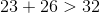.

By the Law of Cosines: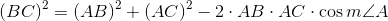Substitute the sidelengths and solve for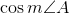: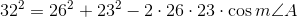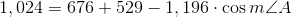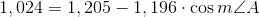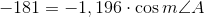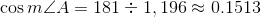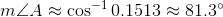### Example Question #2 : Applying The Law Of Cosines

A triangle has sides of length 12, 17, and 22. Of the measures of the three interior angles, which is the greatest of the three?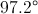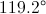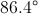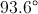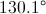Explanation:

We can apply the Law of Cosines to find the measure of this angle, which we will call :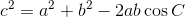The widest angle will be opposite the side of length 22, so we will set: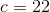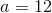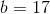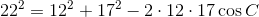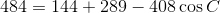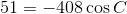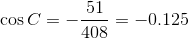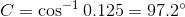### Example Question #3 : Applying The Law Of Cosines

In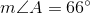,, and. To the nearest tenth, what is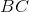?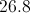A triangle with these characteristics cannot exist.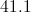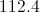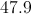Explanation:

By the Law of Cosines:or, equivalently,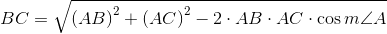Substitute: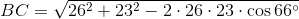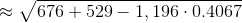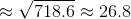### All High School Math Resources